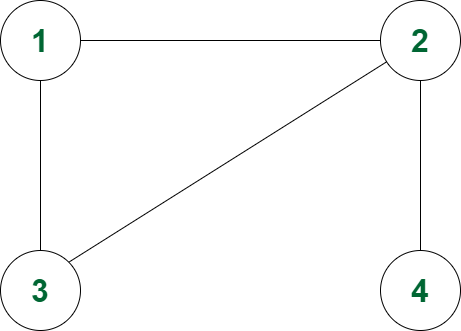# Number of minimum length paths between 1 to N including each node

• Last Updated : 15 Sep, 2021

Given an undirected and unweighted graph of N nodes and M edges, the task is to count the minimum length paths between node 1 to N through each of the nodes. If there is doesn’t exist any such path, then print “-1”.

Note: The path can pass through a node any number of times.

Examples:

Input: N = 4, M= 4, edges = {{1, 2}, {2, 3}, {1, 3}, {2, 4}}
Output: 1 1 1 1
Explanation:Total paths of minimum length from 1 to 4, passing from 1 is 1.
Total paths of minimum length from 1 to 4, passing from 2 is 1.
Total paths of minimum length from 1 to 4, passing from 3 is 1.
Total paths of minimum length from 1 to 4, passing from 4 is 1.

Input: N = 5, M = 5, edges = {{1, 2}, {1, 4}, {1 3}, {2, 5}, {2, 4}}
Output: 1 1 0 1 1

Approach: The given problem can be solved by performing two BFS, one from node 1 excluding node N and another from node N excluding node 1 to find the minimum distance of all the nodes from 1 and N, and the product of both the minimum distances will be the total count of minimum length paths from 1 to N including the node. Follow the steps below to solve the problem:

• Initialize a queue, say queue1 to perform BFS from node 1 and a queue queue2 to perform BFS from node N.
• Initialize arrays, say dist[] to store the shortest distance and ways[] to count the number of ways to reach that node.
• Perform two BFS and perform the following steps in each case:
• Pop from the queue and store node in x and its distance in dis.
• If dist[x] is smaller than dis then continue.
• Traverse the adjacency list of x and for each child y, if dist[y] is greater than dis + 1 then update dist[y] equals dis + 1 and ways[y] equals ways[x]. Otherwise, if dist[y] equals dis +1 then add ways[x] to ways[y].
• Finally, iterate over the range N, and for each node print the count of minimum length paths as ways1[i]*ways2[i].

Below is the implementation of the above approach:

## C++

 `// C++ program for the above approach` `#include ``using` `namespace` `std;``#define ll long long int` `// Function to calculate the distances``// from node 1 to N``void` `countMinDistance(``int` `n, ``int` `m,``                      ``int` `edges[])``{` `    ``// Stores the number of edges``    ``vector g;` `    ``// Storing the edges in vector``    ``for` `(``int` `i = 0; i < m; i++) {``        ``int` `a = edges[i] - 1;``        ``int` `b = edges[i] - 1;``        ``g[a].push_back(b);``        ``g[b].push_back(a);``    ``}` `    ``// Initialize queue``    ``queue > queue1;``    ``queue1.push({ 0, 0 });``    ``vector<``int``> dist(n, 1e9);``    ``vector<``int``> ways1(n, 0);``    ``dist = 0;``    ``ways1 = 1;` `    ``// BFS from 1st node using queue``    ``while` `(!queue1.empty()) {``        ``auto` `up = queue1.front();` `        ``// Pop from queue``        ``queue1.pop();``        ``int` `x = up.first;``        ``int` `dis = up.second;``        ``if` `(dis > dist[x])``            ``continue``;``        ``if` `(x == n - 1)``            ``continue``;` `        ``// Traversing the adjacency list``        ``for` `(ll y : g[x]) {``            ``if` `(dist[y] > dis + 1) {``                ``dist[y] = dis + 1;``                ``ways1[y] = ways1[x];``                ``queue1.push({ y, dis + 1 });``            ``}``            ``else` `if` `(dist[y] == dis + 1) {``                ``ways1[y] += ways1[x];``            ``}``        ``}``    ``}` `    ``// Initialize queue``    ``queue > queue2;``    ``queue2.push({ n - 1, 0 });``    ``vector<``int``> dist1(n, 1e9);``    ``vector<``int``> ways2(n, 0);``    ``dist1[n - 1] = 0;``    ``ways2[n - 1] = 1;` `    ``// BFS from last node``    ``while` `(!queue2.empty()) {``        ``auto` `up = queue2.front();` `        ``// Pop from queue``        ``queue2.pop();``        ``int` `x = up.first;``        ``int` `dis = up.second;``        ``if` `(dis > dist1[x])``            ``continue``;``        ``if` `(x == 0)``            ``continue``;` `        ``// Traverse the adjacency list``        ``for` `(ll y : g[x]) {``            ``if` `(dist1[y] > dis + 1) {``                ``dist1[y] = dis + 1;``                ``ways2[y] = ways2[x];``                ``queue2.push({ y, dis + 1 });``            ``}``            ``else` `if` `(dist1[y] == 1 + dis) {``                ``ways2[y] += ways2[x];``            ``}``        ``}``    ``}` `    ``// Print the count of minimum``    ``// distance``    ``for` `(``int` `i = 0; i < n; i++) {``        ``cout << ways1[i] * ways2[i] << ``" "``;``    ``}``}` `// Driver Code``int` `main()``{``    ``int` `N = 5, M = 5;``    ``int` `edges[M] = {``        ``{ 1, 2 }, { 1, 4 }, { 1, 3 },``        ``{ 2, 5 }, { 2, 4 }``    ``};``    ``countMinDistance(N, M, edges);` `    ``return` `0;``}`

## Python3

 `# Python 3 program for the above approach` `# Function to calculate the distances``# from node 1 to N``def` `countMinDistance(n, m, edges):``    ``# Stores the number of edges``    ``g ``=` `[[] ``for` `i ``in` `range``(``10005``)]` `    ``# Storing the edges in vector``    ``for` `i ``in` `range``(m):``        ``a ``=` `edges[i][``0``] ``-` `1``        ``b ``=` `edges[i][``1``] ``-` `1``        ``g[a].append(b)``        ``g[b].append(a)` `    ``# Initialize queue``    ``queue1 ``=` `[]``    ``queue1.append([``0``, ``0``])``    ``dist ``=` `[``1e9` `for` `i ``in` `range``(n)]``    ``ways1 ``=` `[``0` `for` `i ``in` `range``(n)]``    ``dist[``0``] ``=` `0``    ``ways1[``0``] ``=` `1` `    ``# BFS from 1st node using queue``    ``while` `(``len``(queue1)>``0``):``        ``up ``=` `queue1[``0``]` `        ``# Pop from queue``        ``queue1 ``=` `queue1[:``-``1``]``        ``x ``=` `up[``0``]``        ``dis ``=` `up[``1``]``        ``if` `(dis > dist[x]):``            ``continue``        ``if` `(x ``=``=` `n ``-` `1``):``            ``continue` `        ``# Traversing the adjacency list``        ``for` `y ``in` `g[x]:``            ``if` `(dist[y] > dis ``+` `1``):``                ``dist[y] ``=` `dis ``+` `1``                ``ways1[y] ``=` `ways1[x]``                ``queue1.append([y, dis ``+` `1``])``        ` `            ``elif``(dist[y] ``=``=` `dis ``+` `1``):``                ``ways1[y] ``+``=` `ways1[x]` `    ``# Initialize queue``    ``queue2 ``=` `[]``    ``queue2.append([n ``-` `1``, ``0``])``    ``dist1 ``=` `[``1e9` `for` `i ``in` `range``(n)]``    ``ways2 ``=` `[``0` `for` `i ``in` `range``(n)]``    ``dist1[n ``-` `1``] ``=` `0``    ``ways2[n ``-` `1``] ``=` `1` `    ``# BFS from last node``    ``while``(``len``(queue2)>``0``):``        ``up ``=` `queue2[``0``]` `        ``# Pop from queue``        ``queue2 ``=` `queue2[:``-``1``]``        ``x ``=` `up[``0``]``        ``dis ``=` `up[``1``]``        ``if` `(dis > dist1[x]):``            ``continue``        ``if` `(x ``=``=` `0``):``            ``continue` `        ``# Traverse the adjacency list``        ``for` `y ``in` `g[x]:``            ``if` `(dist1[y] > dis ``+` `1``):``                ``dist1[y] ``=` `dis ``+` `1``                ``ways2[y] ``=` `ways2[x]``                ``queue2.append([y, dis ``+` `1``])` `            ``elif``(dist1[y] ``=``=` `1` `+` `dis):``                ``ways2[y] ``+``=` `ways2[x]` `    ``# Print the count of minimum``    ``# distance``    ``ways1[n``-``1``] ``=` `1``    ``ways2[n``-``1``] ``=` `1``    ``for` `i ``in` `range``(n):``        ``print``(ways1[i] ``*` `ways2[i],end ``=` `" "``)``    `  `# Driver Code``if` `__name__ ``=``=` `'__main__'``:``    ``N ``=` `5``    ``M ``=` `5``    ``edges ``=` `[[``1``, ``2``],[``1``, ``4``],[``1``, ``3``],[``2``, ``5``],[``2``, ``4``]]``    ``countMinDistance(N, M, edges)``    ` `    ``# This code is contributed by SURENDRA_GANGWAR.`

## Javascript

 ``
Output:
`1 1 0 1 1`

Time Complexity: O(N + M)
Auxiliary Space: O(N)

My Personal Notes arrow_drop_up